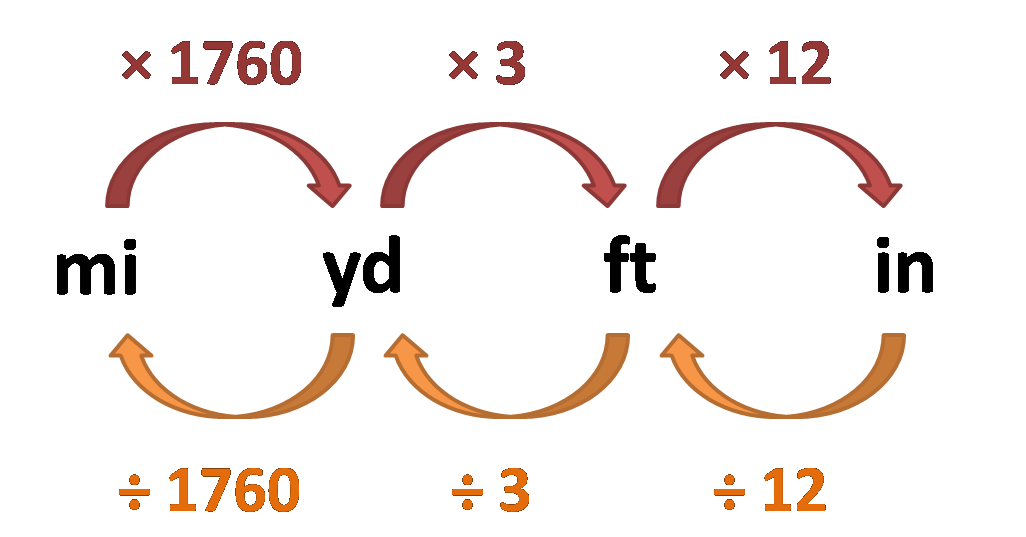# Yard to Mile Converter

Created by Julia Żuławińska
Reviewed by Komal Rafay
Last updated: May 03, 2023

The yard to mile converter is here for you to help you calculate miles from yards. It also works in reverse, and you can use it as a mi to yd converter. Keep reading to learn how to do these calculations on your own and to find similar tools.

## Conversion chart - miles to yards

The below conversion chart shows how to convert miles to yards to feet to inches. As you see, it's fairly easy - the tricky part is remembering the conversion factors.

If you know a distance in miles, you need to multiply it by 1760 to get yards. From there, you can multiply it times 3 to get feet. Then, multiply it times 12 to get inches. You can also go the other way and divide a number by 12, 3, or 1760.## Other tools similar to mi to yd converter

Our website is full of useful calculators. Here are some of them that deal with length conversion:

## FAQ

### What is the conversion factor used to convert miles to yards?

The conversion factor in calculating miles to yards is 1760.
1 mile = 1760 yards
So, to find the distance in miles, you need to divide the yards by 1760:
miles = yards / 1760
To find the distance in yards, multiply the miles times 1760:
yards = miles ×1760
To save time, our yard to mile converter is always available.

### How many miles is 500 yards?

500 yards equals around 0.3 miles or, more precisely, 0.2841 miles. To convert miles and yards, you need to:

• multiply the distance in miles times 1760 to get yards, and
• divide the distance in yards by 1760 to get miles.
Julia Żuławińska
yd
mi
People also viewed…

### Coffee kick

A long night of studying? Or maybe you're on a deadline? The coffee kick calculator will tell you when and how much caffeine you need to stay alert after not sleeping enough 😀☕ Check out the graph below!

### Cups to quarts converter

The cups to quarts converter is your one stop tool for a quick and convenient volumetric conversion.

### Gallons to pounds

Use our gallons to pounds calculator to calculate the weight of gallons of water or other liquids.

### Titration

Use our titration calculator to determine the molarity of your solution.